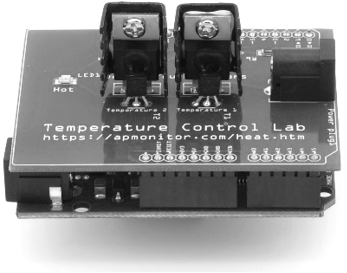## Quiz on Linear Programming1. Which of the following describes the simplex method for linear programming?

A. Picking a point in the feasible region and following the gradient to the maximum or minimum
Incorrect. This describes the interior point method
B. Find and compare intersections of constraints within the feasible region and compare to find the maximum or minimum
Correct. The simplex method is an effective strategy for linear programming problems where the solution is always at the intersection of constraints. The interior point method takes a different approach by starting with a feasible initial guess and iteratively reducing the barrier term until reaching the solution.
C. Choose a set of feasible points and iteratively move each towards its local best known position and global best known position
Incorrect. This is a description of particle swarm optimization
D. Cycle through multiple generations of trial solutions where a fitness function determines the best solution
Incorrect. This is a description of a genetic algorithm

2. The soft drink production exercise is revisited, but from the perspective of a consumer trying to minimize cost. The consumer must buy at least 2 units of Product 1 and 3 units of Product 2. Change the lower bounds for x1 and x2 and change the Maximize to Minimize. Solve the problem to find the optimal cost.

# currently producer optimization, change to consumer optimization
from gekko import GEKKO
m = GEKKO()
x1 = m.Var(lb=0, ub=5) # Product 1
x2 = m.Var(lb=0, ub=4) # Product 2
m.Maximize(100*x1+125*x2) # Profit function
m.Equation(3*x1+6*x2<=30) # Units of A
m.Equation(8*x1+4*x2<=44) # Units of B
m.solve(disp=False)
p1 = x1.value; p2 = x2.value
print ('Product 1 (x1): ' + str(p1))
print ('Product 2 (x2): ' + str(p2))
print ('Profit        : ' + str(100*p1+125*p2))
A. 775
Incorrect. This is the maximum. Switch to m.Minimize().
B. 575
Correct. Lower bounds changed to 2 and 3 and Maximize changed to Minimize
from gekko import GEKKO
m = GEKKO()
x1 = m.Var(lb=2, ub=5) # Product 1
x2 = m.Var(lb=3, ub=4) # Product 2
m.Minimize(100*x1+125*x2) # Profit function
m.Equation(3*x1+6*x2<=30) # Units of A
m.Equation(8*x1+4*x2<=44) # Units of B
m.solve(disp=False)
p1 = x1.value; p2 = x2.value
print ('Product 1 (x1): ' + str(p1))
print ('Product 2 (x2): ' + str(p2))
print ('Profit        : ' + str(100*p1+125*p2))
C. 570
Incorrect. Change the lower bounds and change Maximize to Minimize
D. 580
Incorrect. Change the lower bounds and change Maximize to Minimize

3. Ingredients (A and B) are used to make 2 products (Products 1 and 2). Production requires:

• 1 unit of A and 4 units of B for Product 1
• 2 units of A and 6 units of B for Product 2

The available supply is A = 20 and B = 40. Choose the Python code that defines the E matrix and b vector for the supply constraints in this linear programming problem.

$$\mathrm{minimize} \quad c\,x$$ $$\quad\quad\quad\quad E \, x<b$$

A. E = [2,4], [1,6] and b = [20, 40]
Incorrect. Missing the matrix brackets and incorrect numbers
B. E = [ [1,2], [4,6] ] and b = [40, 20]
Incorrect. The values in vector b need to be switched
C. E = [ [1,2], [4,6] ] and b = [20, 40]
Correct. The two inequality equations are for supply limitations for A: x_1 + 2 x_2 \le 40 and for B: 6 x_1 + 4 x_2 \le 20
D. E = [1,1, 2,2], [1,6] and b = [20, 40]

4. Select the code that expresses the matrix as a sparse matrix in coordinate index form with row, column, value rows with index-1 that starts at 1 instead of the typical Python convention of 0.

$$E = \begin{bmatrix} 2 & 4 \\ 1 & 0 \end{bmatrix}$$

A. E_sparse = [ [1,1,2,2],[1,2,1,2],[2,4,1,0] ]
Incorrect. The zeros entries are omitted for a sparse matrix
B. E_sparse = [ [1,1,2],[1,2,1],[2,4,1] ]
Correct. The index and values for the three non-zero matrix entries with index-1.
C. E_sparse = [ [1,2,1,2],[1,2,1,2],[2,4,1,0] ]
Incorrect. The three rows are row, column, value. The index is incorrect and zeros are omitted in a sparse matrix.
D. E_sparse = [ [1,1,2],[1,2,4],[2,1,1] ]
Incorrect. This is the transpose of the correct answer.